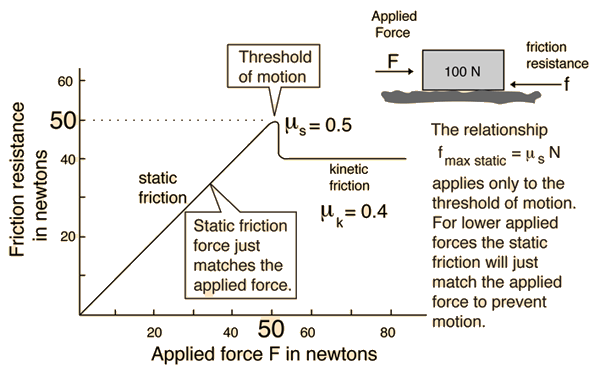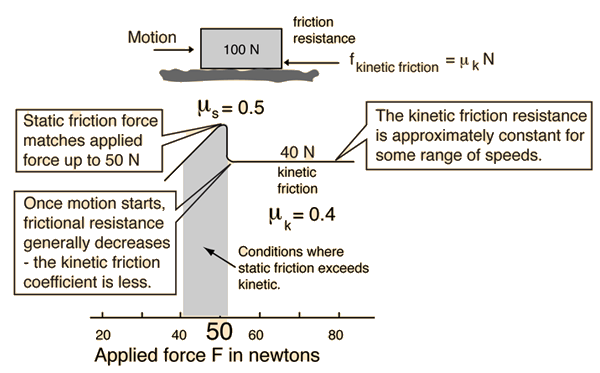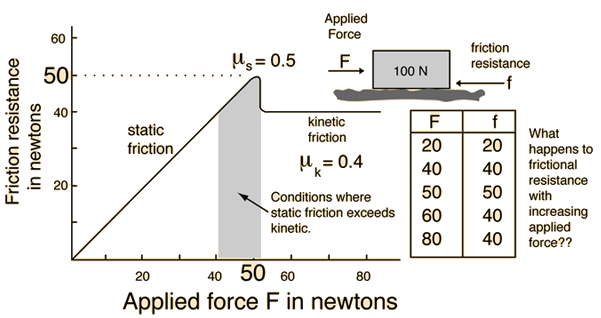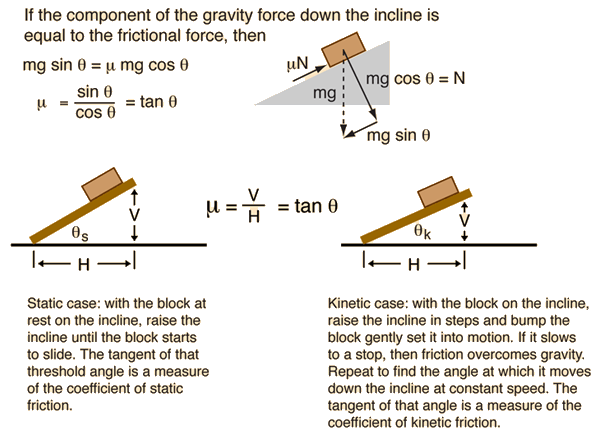# Static Friction

Static frictional forces from the interlocking of the irregularities of two surfaces will increase to prevent any relative motion up until some limit where motion occurs. It is that threshold of motion which is characterized by the coefficient of static friction. The coefficient of static friction is typically larger than the coefficient of kinetic friction.In making a distinction between static and kinetic coefficients of friction, we are dealing with an aspect of "real world" common experience with a phenomenon which cannot be simply characterized. The difference between static and kinetic coefficients obtained in simple experiments like wooden blocks sliding on wooden inclines roughly follows the model depicted in the friction plot from which the illustration above is taken. This difference may arise from irregularities, surface contaminants, etc. which defy precise description. When such experiments are carried out with smooth metal blocks which are carefully cleaned, the difference between static and kinetic coefficients tends to disappear. When coefficients of friction are quoted for specific surface combinations are quoted, it is the kinetic coefficient which is generally quoted since it is the more reliable number.

Index

Friction concepts

 HyperPhysics***** Mechanics R Nave
Go Back

# Kinetic Friction

When two surfaces are moving with respect to one another, the frictional resistance is almost constant over a wide range of low speeds, and in the standard model of friction the frictional force is described by the relationship below. The coefficient is typically less than the coefficient of static friction, reflecting the common experience that it is easier to keep something in motion across a horizontal surface than to start it in motion from rest.Index

Friction concepts

 HyperPhysics***** Mechanics R Nave
Go Back

# Friction Plot

Static friction resistance will match the applied force up until the threshold of motion. Then the kinetic frictional resistance stays about constant. This plot illustrates the standard model of friction.The above plot, though representing a simplistic view of friction, agrees fairly well with the results of simple experiments with wooden blocks on wooden inclines. The experimental procedure described below equates the vector component of the weight down the incline to the coefficient of friction times the normal force produced by the weight on the incline.Having taken a large number of students through this experiment, I can report that the coefficient of static friction obtained is almost always greater than the coefficient of kinetic friction. Typical results for the woods I have used are 0.4 for the static coefficient and 0.3 for the kinetic coefficient.

When carefully standardized surfaces are used to measure the friction coefficients, the difference between static and kinetic coefficients tends to disappear, indicating that the difference may have to do with irregular surfaces, impurities, or other factors which can be frustratingly non-reproducible. To quote a view counter to the above model of friction:
"Many people believe that the friction to be overcome to get something started (static friction) exceeds the force required to keep it sliding (sliding friction), but with dry metals it is very hard to show any difference. The opinion probably arises from experiences where small bits of oil or lubricant are present, or where blocks, for example, are supported by springs or other flexible supports so that they appear to bind." R. P. Feynman, R. P. Leighton, and M. Sands, The Feynman Lectures on Physics, Vol. I, p. 12-5, Addison-Wesley, 1964.

Index

Friction concepts

Reference
Jones & Childers, 2nd Ed.
Sec 4.8

 HyperPhysics***** Mechanics R Nave
Go Back

# Rolling Friction

A rolling wheel requires a certain amount of friction so that the point of contact of the wheel with the surface will not slip. The amount of traction which can be obtained for an auto tire is determined by the coefficient of static friction between the tire and the road. If the wheel is locked and sliding, the force of friction is determined by the coefficient of kinetic friction and is usually significantly less.

Assuming that a wheel is rolling without slipping, the surface friction does no work against the motion of the wheel and no energy is lost at that point. However, there is some loss of energy and some deceleration from friction for any real wheel, and this is sometimes referred to as rolling friction. It is partly friction at the axle and can be partly due to flexing of the wheel which will dissipate some energy. Figures of 0.02 to 0.06 have been reported as effective coefficients of rolling friction for automobile tires, compared to about 0.8 for the maximum static friction coefficient between the tire and the road.

Index

Friction concepts

 HyperPhysics***** Mechanics R Nave
Go Back